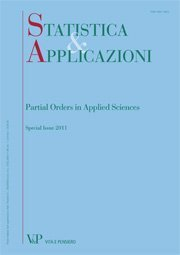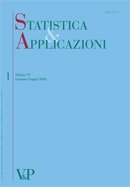Your browser does not support JavaScript!

Search results

Maximum likelihood estimator of the shape parameter of the weibull distribution using ranked set sampling digitalformat:Article| STATISTICA & APPLICAZIONI - 2013 - 2
Year: 2014
In this paper, we will consider the (maximum likelihood estimators) MLEs of the shape parameter p of the Weibull distribution...
Estimation of the variance for logistic distribution under ranked set sampling and simple random sampling: a comparative study
Free
digitalformat:Article| STATISTICA & APPLICAZIONI - 2012 - 2
Year: 2012
SUMMARY The logistic distribution is applicable in many area of research. In this study, several estimators of the variance when the location parameter is known and unknown are considered when data are gathered under simple random sampling (SRS) and ranked set sampling (RSS). For some estimators considered, the bias and mean square error (MSE) are not gotten in closed form. Using Monte Carlo simulations, comparison of these estimators is made based on biases, MSE and efficiency. When the estimators are compared, it is found that estimators based on maximum likelihood method are more efficient than other estimators considered, under both SRS and RSS. However, estimators based on RSS have more advantages over those based on SRS. Keywords: Logistic Distribution, Ranked set Sampling, Simple Random Sampling, Variance, Estimations.
Estimating the normal population with known coefficient of variation using the optimal ranked set sampling scheme
Free
digitalformat:Article| STATISTICA & APPLICAZIONI - 2012 - 1
Year: 2012
SUMMARY In the ranked set sampling with set size equal to m, there are mm different possible sampling schemes. The aim of this paper is to characterize the optimal sampling scheme via the Fisher information numbers for normal distribution with known coefficient of variation. Moreover, we use the optimal sampling schemes to obtain unbiased estimators for the mean. Also we compare the estimators to their counterparts under the McIntyre’s ranked set sampling scheme. We show that the MLE under optimal sampling scheme is asymptotically more efficient than its counterpart under McIntyre’s RSS. Keywords: Ranked Set Sampling, Fisher Information, Maximum Likelihood, Normal Distribution, Coefficient of Variation.
Median ranked set sampling for polynomial regression
Free
digitalformat:Article| STATISTICA & APPLICAZIONI - 2011 - 1
Year: 2011
SUMMARY The purpose of this article is to study the ploynomial linear regression model under the median ranked set sampling (MRSS) scheme introduced by Muttlak (1997). If the response variable can be more easily ranked than quantified, then we use the MRSS to collect data by ranking on the response variable. We obtain estimators and confidence intervals for the polynomial regression parameters under MRSS when the errors have a symmetric distribution. We also show that the least square estimators, under MRSS, are more efficient than their SRS counterparts and give illustrating examples. Keywords: Median Ranked Set Sample, Polynomial Regression.
Estimation of  = P(X>Y) using ranked set sampling
Free
digitalformat:Article| STATISTICA & APPLICAZIONI - 2010 - 2
Year: 2010
SUMMARY Nonparametric-type estimation of  ¼ PðX > YÞ, based on ranked set sampling (RSS) technique with concomitant random variable, is considered. The maximum likelihood estimation (MLE) of  is also considered using RSS and median ranked set sampling (MRSS) techniques with concomitant random variable. The estimators obtained are compared to their counterparts based on simple random sampling (SRS) using bias and mean square error (MSE). It appears that the suggested nonparametric estimator based on RSS is more efficient than that based on SRS and the MLE based on MRSS is more efficient than the MLE based on RSS which is in turn more efficient than the MLE based on SRS. Keywords: Simple Random Sampling, Ranked Set Sampling, Median Ranked Set Sampling, Nonparametric Estimation; Maximum Likelihood Estimation, Mean Square Error. RIASSUNTO La stima non parametrica di  ¼ PðX > YÞ basata sulla tecnica del Ranked Set Sampling (RSS) con variabile concomitante e` considerata preliminarmente. Successivamente viene trattata la Stima di Massima Verosimiglianza (MLE) di  nel caso Normale usando sia RSS sia il Median Ranked Set Sampling (MRSS) con variabile concomitante. Gli stimatori ottenuti sono confrontati con quelli basati sul Simple Random Sample (SRS) usando il bias e l’errore quadratico medio (MSE). Emerge che lo stimatore non parametrico proposto basato su RSS e`piu` efficiente di quello basato su SRS e lo stimatore MLE basato su MRSS e`piu` efficiente di quello MLE basato su RSS che a sua volta risulta piu` efficiente di quello MLE basato su SRS.
Moving extreme ranked set sampling for simple linear regression
Free
digitalformat:Article| STATISTICA & APPLICAZIONI - 2009 - 2
Year: 2009
The moving extreme ranked set sampling, introduced by Alodat and Al-Saleh (2001), is a modification of the well known ranked set sampling approach that was proposed by McIntyre (1952). In this paper, we suggest new estimators for the simple linear regression parameters under the moving extreme ranked set sampling scheme. Moreover, we show that the proposed estimators are more efficient than their counterparts using the simple random sampling approach. We illustrate our ideas and thoughts via simulation and data analysis and conduct a comparison between our approach and the traditional ones. Keywords: Moving ranked set sampling, Ranked set sampling, Simple linear regression.